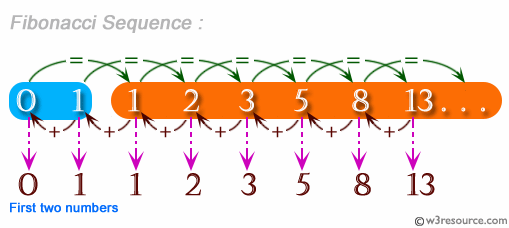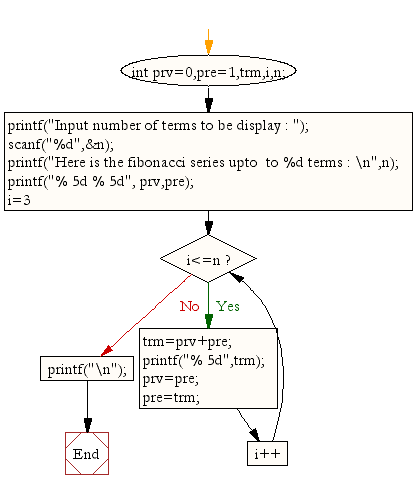﻿ C Program: Display the first n terms of fibonacci series - w3resource# C Exercises: Display the first n terms of Fibonacci series

## C For Loop: Exercise-35 with Solution

Write a program in C to display the first n terms of Fibonacci series. The series is as follows:
Fibonacci series 0 1 2 3 5 8 13 .....

Pictorial Presentation:Sample Solution:

C Code:

``````#include <stdio.h>

void main()
{
int prv=0,pre=1,trm,i,n;
printf("Input number of terms to  display : ");
scanf("%d",&n);
printf("Here is the Fibonacci series upto  to %d terms : \n",n);
printf("% 5d % 5d", prv,pre);

for(i=3;i<=n;i++)
{
trm=prv+pre;
printf("% 5d",trm);
prv=pre;
pre=trm;
}
printf("\n");
}
```
```

Sample Output:

```Input number of terms to  display : 10
Here is the Fibonacci series upto  to 10 terms :
0     1    1    2    3    5    8   13   21   34
```

Flowchart:C Programming Code Editor:

Improve this sample solution and post your code through Disqus.

What is the difficulty level of this exercise?

Test your Programming skills with w3resource's quiz.

﻿

## C Programming: Tips of the Day

Is there a way to specify how many characters of a string to print out using printf()?

The basic way is:

`printf ("Here are the first 8 chars: %.8s\n", "A string that is more than 8 chars");`

The other, often more useful, way is:

`printf ("Here are the first %d chars: %.*s\n", 8, 8, "A string that is more than 8 chars");`

Here, you specify the length as an int argument to printf(), which treats the '*' in the format as a request to get the length from an argument.

You can also use the notation:

```printf ("Here are the first 8 chars: %*.*s\n",
8, 8, "A string that is more than 8 chars");```

This is also analogous to the "%8.8s" notation, but again allows you to specify the minimum and maximum lengths at runtime - more realistically in a scenario like:

`printf("Data: %*.*s Other info: %d\n", minlen, maxlen, string, info);`

The POSIX specification for printf() defines these mechanism

Ref : https://bit.ly/3u32GyO